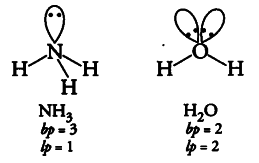# Although geometries of \${ NH }_{ 3 }\$ and \$H _{ 2 }O\$

Although geometries of \${ NH }_{ 3 }\$ and
\$H _{ 2 }O\$ molecules are distorted tetrahedral, bond angle in water is less than that of ammonia. Discuss.In H _{ 2 }O molecule, there is lone pair-lone pair repulsion due the presence of two lone pairs of electrons while in
{ NH }_{ 4 } molecule there found only lone pair-bond pair repulsion. According to VSEPR theory, the former one is more stronger and hence, the bond angle in water is less than that of ammonia